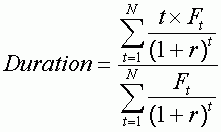Duration of a bond

Around the formula...

For any fixed-income debt portfolio or security, there is a period over which:

• the loss on the reinvestment of coupons will be offset by the capital gain on the sale of the bond if interest rates decline; and
• the gain on the reinvestment of coupons will be offset by the capital loss on the sale of the bond if interest rates rise.

All in all, once this period ends, the overall value of the portfolio (i.e. bonds plus reinvested coupons) is the same, and the investors will have achieved a return on investment identical to the yield to maturity indicated when the bond was issued. In such circumstances, the portfolio is said to be immunised, i.e. it is protected against the risk of fluctuations in interest rates (capital risk and coupon reinvestment risk). This time period is known as the duration of a bond.

From a mathematical standpoint, duration is calculated as follow :Duration is similar to the discounted average life of all the cash flows of a bond (i.e. interest and capital). The numerator comprises the discounted cash flows weighted by the number of years to maturity, while the denominator reflects the present value of the debt.